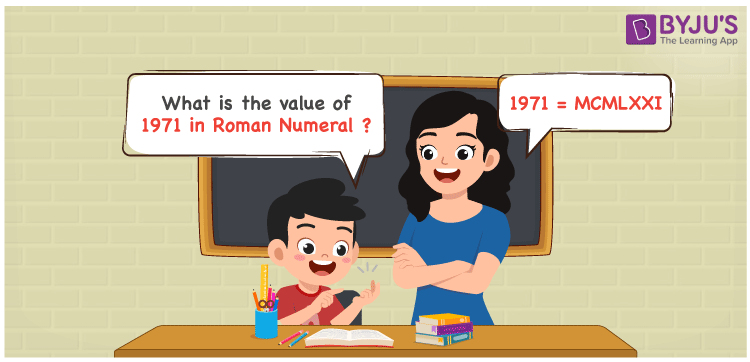# 1971 in Roman Numerals

1971 in Roman Numerals is MCMLXXI. By going through this article, students will get a proper understanding on the roman numerals conversion which is important to attain remarkable grades. The steps used to expand the number and the conversion to roman numerals can be memorized well in this article. Hence, 1971 can be written as MCMLXXI in roman numerals.

 Number Roman Numeral 1971 MCMLXXI## How to Write 1971 in Roman Numerals?

This section gives you a clear understanding of how 1971 can be written in roman numerals.

1971 = 1000 + (1000 – 100) + 50 + 10 + 10 + 1

1971 = M + (M – C) + L + X + X + I

1971 = MCMLXXI

## Video Lesson on Roman Numerals## Frequently Asked Questions on 1971 in Roman Numerals

Q1

### Find the value of 1971 in Roman Numerals.

In the number 1971,
Thousands = 1000 = M
Hundreds = 900 = CM
Tens = 70 = LXX
Ones = 1 = I
So the number = 1000 + 900 + 70 + 1 = MCMLXXI
Q2

### Determine 2000 – 29.

We know that
2000 in Roman Numerals = MM
29 in Roman Numerals = XXIX
As 2000 – 29 = 1971
So 2000 – 29 is 1971 which is written as MCMLXXI.
Q3

### How to write 1971 in Roman Numerals?

1971 can be written in Roman Numerals by considering each digit based on its place value.